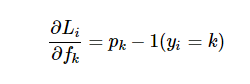# Loss Functions in Deep Learning

I completed Calculus AB and BC during high school because I did not want to deal with mathematics during college despite loving the subject Somehow the general thought that math is hard seeped into me and I diverged from studying the subject. Little did I know that my journey into the field of deep learning would bring you right back to the driving derivatives that I enjoyed so much!

This post is going to be a quick overview of loss functions used for different deep learning models and the math behind them.

# Loss Function

Let’s say we are training an object detection model and the final output is going to look like this; “output = C + 1 + 4” C is the different classes we are going to classify the object into 1 is the confidence score of whether the object belonging to that class exists in the box 4 is the four coordinates for the object

Majority of this post is going to talk about the probability output for C. Let’s say we have 3 classes to classify between, Car, Pedestrian, & Truck. The network is going to output, “[5, 4, 2]”. In order to simplify this into a probability that an object belongs to a certain class, we are going to use a Softmax function.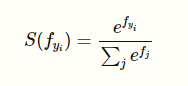What the function does is it squashes a vector of size K between 0 and 1 where K is the number of classes. So, the softmax function in our case would squash [5, 4, 2] between 0 and 1 such that the total of the output vector would equal 1. For example, the output of the application of softmax on the first class output would be;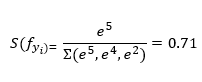In the same way, we are going to compute the probabilities for all the classes that we think the object in the bounding box belongs to, [0.71, 0.26, 0.03]. In the same way, we could use a log_softmax function to get the predicted class’s log probabilities;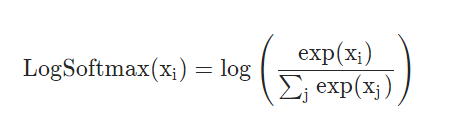This function returns a tensor of the same dimension and shape as the input with values in the range [-inf, 0]. The log Softmax function outputs log likelihood of the softmax function, which penalizes larger error because of the exponential nature of the log softmax function. But even more important than the log likelihood is the negative log likelihood;The softmax function inside the brackets is going to provide us with normalized probabilities after doing the whole arithmetic as explained above. The reason we are using negative log likelihood is because we are trying to determine which class is correct and when the network predict a high correct class probability, the expression for Loss(i) is low and it is very high when the predicted class probability for correct class is low. This makes intuitive sense because of how the negative log likelihood function works;When we input a very small probability for the correct class label, the loss function is going toward positive infinity but when the probability for correct class label is high i.e. it goes towards 1, the loss function goes towards zero. Now we are going to look at how Loss function works,

## Cross-Entropy Loss

The Cross-entropy loss makes use of Softmax Classifier as well as regularization loss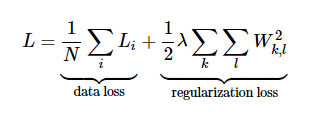We first compute the correct log probabilities in the first half of the equation and divide them by the total number of examples. The regularization loss can be computed by multiplying regularization strength lambda with sum of W matrix squared. The final loss is going to add the data loss as well as the regularization loss.

Now that we have a way to understand the loss, we are going to look at how to optimize that loss to be the minimum via gradient descent.

In gradient descent, we are trying to minimize the loss by evaluating the gradient of the loss function with respect to parameters. In doing so, we will know how should we change the parameters to decrease the loss;